Ordering MCQ - 5

# Ordering MCQ - 5 - Bank Exams

Test Description

## 20 Questions MCQ Test Reasoning Aptitude for Competitive Examinations - Ordering MCQ - 5

Ordering MCQ - 5 for Bank Exams 2023 is part of Reasoning Aptitude for Competitive Examinations preparation. The Ordering MCQ - 5 questions and answers have been prepared according to the Bank Exams exam syllabus.The Ordering MCQ - 5 MCQs are made for Bank Exams 2023 Exam. Find important definitions, questions, notes, meanings, examples, exercises, MCQs and online tests for Ordering MCQ - 5 below.
Solutions of Ordering MCQ - 5 questions in English are available as part of our Reasoning Aptitude for Competitive Examinations for Bank Exams & Ordering MCQ - 5 solutions in Hindi for Reasoning Aptitude for Competitive Examinations course. Download more important topics, notes, lectures and mock test series for Bank Exams Exam by signing up for free. Attempt Ordering MCQ - 5 | 20 questions in 15 minutes | Mock test for Bank Exams preparation | Free important questions MCQ to study Reasoning Aptitude for Competitive Examinations for Bank Exams Exam | Download free PDF with solutions
 1 Crore+ students have signed up on EduRev. Have you?
Ordering MCQ - 5 - Question 1

### Six students P, Q, R, S T and V are the top six rankers of the class. No two persons got the same rank. The student who got the highest marks is given rank 1 and the student who got the least marks is given rank 6. Q got less marks than both R and U. P got more marks than T but less than S. Q got the second least rank and U got the second highest rank, R got less marks than P. Q.  ______got the 3rd rank.

Detailed Solution for Ordering MCQ - 5 - Question 1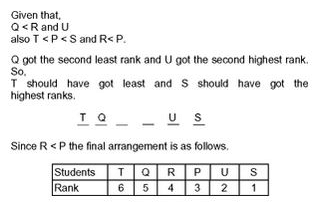Ordering MCQ - 5 - Question 2

### Six students P, Q, R, S T and V are the top six rankers of the class. No two persons got the same rank. The student who got the highest marks is given rank 1 and the student who got the least marks is given rank 6. Q got less marks than both R and U. P got more marks than T but less than S. Q got the second least rank and U got the second highest rank, R got less marks than P. Q. __________got the 6th rank.

Ordering MCQ - 5 - Question 3

### Directions for questions. These questions are based on the following information. Six persons A, B, C, D, E and F are comparing their weights and each of them is having a different weight. D is heavier than only two persons. E is the only person heavier than F and lighter than D. C’s weight is less than only B’s weight. The third heaviest weight is 100kgs and the fifth heaviest weight is 60kgs. Q. What is the possible weight of D?

Detailed Solution for Ordering MCQ - 5 - Question 3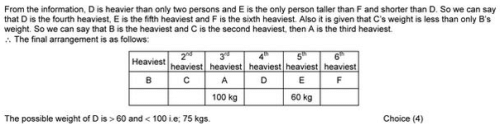Ordering MCQ - 5 - Question 4

Directions for questions. These questions are based on the following information. Six persons A, B, C, D, E and F are comparing their weights and each of them is having a different weight. D is heavier than only two persons. E is the only person heavier than F and lighter than D. C’s weight is less than only B’s weight. The third heaviest weight is 100kgs and the fifth heaviest weight is 60kgs.

Q.

Who is the third heaviest

Ordering MCQ - 5 - Question 5

Directions for questions. These questions are based on the following information. Six persons A, B, C, D, E and F are comparing their weights and each of them is having a different weight. D is heavier than only two persons. E is the only person heavier than F and lighter than D. C’s weight is less than only B’s weight. The third heaviest weight is 100kgs and the fifth heaviest weight is 60kgs.

Q.

If the heaviest weight is 30kgs more than A’s weight, then what is the possible weight of C?

Ordering MCQ - 5 - Question 6

Directions for questions : These questions are based on the following information. Six persons P, Q, R, S, T and U are comparing their heights. No two persons are of the same height. U is taller than P, who is taller than S and R. Only two persons are shorter than T, but neither of them is R. S is not the shortest person. R is 170 cm tall.

Q.

if S is 160 cm tall,then height of T is_______

Ordering MCQ - 5 - Question 7

Directions for questions : These questions are based on the following information. Six persons P, Q, R, S, T and U are comparing their heights. No two persons are of the same height. U is taller than P, who is taller than S and R. Only two persons are shorter than T, but neither of them is R. S is not the shortest person. R is 170 cm tall.

Q.

_____is the 3rd tallest person.

Ordering MCQ - 5 - Question 8

Directions for questions : These questions are based on the following information. Six persons P, Q, R, S, T, and U participated in a running race and each of them completed the race in different positions. S finished the race after only two other persons finished the race. U is the only person to finish the race before P and after R. T is not the first person to finish the race.

Q.

______ finished the race in 1st position.

Detailed Solution for Ordering MCQ - 5 - Question 8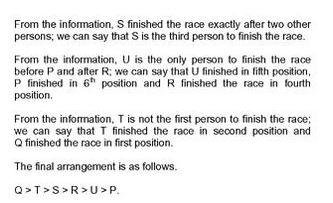Ordering MCQ - 5 - Question 9

Directions for questions : These questions are based on the following information. Six persons P, Q, R, S, T, and U participated in a running race and each of them completed the race in different positions. S finished the race after only two other persons finished the race. U is the only person to finish the race before P and after R. T is not the first person to finish the race.

Q.

If the speed of S is 18 km/hr and the speed of Q is 24 km/hr, then what can the speed of T be?

Ordering MCQ - 5 - Question 10

Aman starts from his house and walks 2 km east up to his class and turns southwards and walks 1 km up to his friend’s house. At the friend’s house, he turns to east and walks 2 km up to his school. He then turns northwards and walks 4 km to the garden. How far is he from his house?

Detailed Solution for Ordering MCQ - 5 - Question 10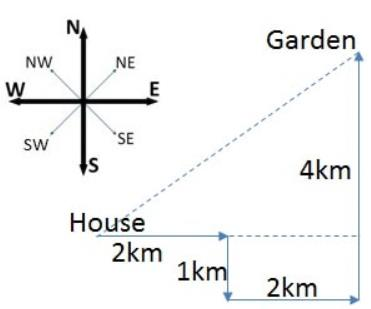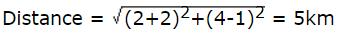Ordering MCQ - 5 - Question 11

Kajal ranked 10 from the top and 26 from the bottom among those who passes an examination. 4 students didn’t attend the exam and 6 students failed in the exam.How many students were in the class ?

Ordering MCQ - 5 - Question 12

In a row of students facing north, Suresh is 8 to the left of Prem, who is 12 from the right end. Manoj is 13 from the left end and third to the right of the Suresh.Find the total no of students in the row ?

Ordering MCQ - 5 - Question 13

In a row of people A is 12 from the left end and B is 8 from the right end. When they exchange their position, A becomes 22 from the left end, What will be the B’s position from the right end ?

Ordering MCQ - 5 - Question 14

There are 30 children in a row. P is 12 to the left of Q and 18 from the right end of the row. What will be the Q’s position from the left end ?

Ordering MCQ - 5 - Question 15

In a class of 42 children, Sakthi rank is 7 from the top.Mahesh is 4 ranks below Sakthi, What is Mahesh rank from the bottom ?

Ordering MCQ - 5 - Question 16

Group of friends are sitting in a row facing North. Jo is 5 to the left of Ashu who is 8 to the right of Diya. How many persons sitting between Jo and Diya ?

Ordering MCQ - 5 - Question 17

In a row of 25 people,Harini is 8 from the right end and Thara is 4 from the left end.How many students are there between Harini and Thara ?

Ordering MCQ - 5 - Question 18

In a row of children facing North Vishnu is 12 from left. When shifted to his right by four places, he becomes 18 from the right end of the row. How many children in the row ?

Ordering MCQ - 5 - Question 19

In a row of boys, Raj is 12 from the left and Mohan is 16 from the right.If they interchange their position. Mohan becomes 12 from the left. How many girls are there in the row ?

Ordering MCQ - 5 - Question 20

A is 11 from the left end in a row. B is 10 from the right end and 12 from the left end. How many children are toward the right of A in that row ?

## Reasoning Aptitude for Competitive Examinations

11 videos|20 docs|171 tests
Information about Ordering MCQ - 5 Page
In this test you can find the Exam questions for Ordering MCQ - 5 solved & explained in the simplest way possible. Besides giving Questions and answers for Ordering MCQ - 5, EduRev gives you an ample number of Online tests for practice

## Reasoning Aptitude for Competitive Examinations

11 videos|20 docs|171 tests